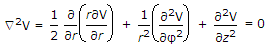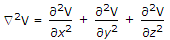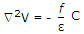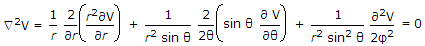# Electronics and Communication Engineering - Electromagnetic Field Theory

11.

Which of the following is abnormal variation in ionosphere?

 A. Diurnal variation B. Seasonal variation C. Ionospheric storms D. None of the above

Answer: Option C

Explanation:

No answer description available for this question. Let us discuss.

12.

Laplace equation in cylindrical co-ordinates is given by

 A.B.C.D.Answer: Option A

Explanation:

No answer description available for this question. Let us discuss.

13.

Troposphere is the upper part of the Earth's atmosphere which absorbs large quantities of Sun's radiations.

 A. True B. False

Answer: Option B

Explanation:

No answer description available for this question. Let us discuss.

14.

For resonant long wire antenna 'n' half wavelength long in free space, if 'n' is increased then inclination of the major lobe with wire axis will __________ .

 A. increases B. decreases C. remains same D. none

Answer: Option B

Explanation:

No answer description available for this question. Let us discuss.

15.

The maximum effective aperture of λ/2 (half-wave) antenna is

 A. 0.013 λ2 B. 0.13 λ2 C. 1.3 λ2 D. 13 λ2

Answer: Option B

Explanation:

No answer description available for this question. Let us discuss.

#### Current Affairs 2021

Interview Questions and Answers